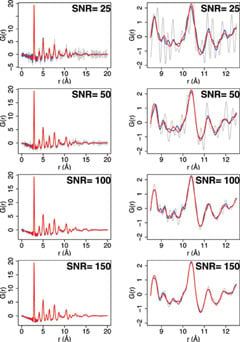•# Mitigation of errors in pair distribution function analysis of nanoparticles

## J. Appl. Cryst. (2011). 44, 788–797 (doi.org/10.1107/S0021889811014749)PDFs (grey) generated using total scattering data representing counting statistics noise, with the signal-to-noise ratio indicated in the upper right corner of each plot. Smoothing the total scattering data results in approximations (blue) to the PDF (red) obtained from noise-free scattering data. The right plots represent selected ranges from the left plots.

This paper discusses procedures for minimizing systematic errors in atomic pair distribution function (PDF) calculations for nanoparticles. The use of a Fourier-transformed small-angle scattering data set to model a baseline in the PDF calculated from wide-angle scattering data is shown to be a promising means of eliminating errors due to use of analytical baseline functions. It is also shown that smoothing noise due to counting statistics in reciprocal-space data can improve the precision of parameter estimates obtained from PDF analysis thus facilitating selection of the correct structural model.

K. Mullen and I. Levin